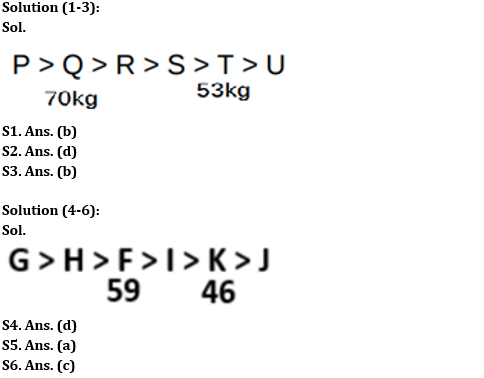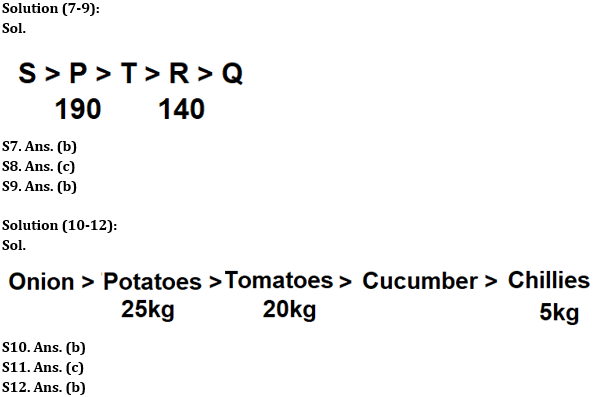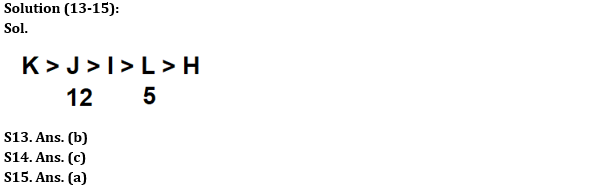Latest Banking jobs   »

# Reasoning Ability Quiz For IBPS RRB PO Prelims 2022- 7th June

Directions (1-3): Study the following information carefully and answer the given questions.
Amongst six friends – P, Q, R, S, T, and U each have different weights. Three persons are lighter than R. S is heavier than U but lighter than P. S is lighter than R. Q is heavier than R but not the heaviest. T is just heavier than U. The weight of the second lightest person is 53 kg and the second heaviest person is 70 kg.

Q1. Who amongst the following is the heaviest?
(a) Either P or T
(b) P
(c) T
(d) S
(e) None of these

Q2. What may be the possible weight of R?
(a) 77kg
(b) 35kg
(c) 48kg
(d) 62kg
(e) None of these

Q3. How many people are heavier than Q?
(a) Three
(b) One
(c) Two
(d) None
(e) Four

Directions (4-6): Study the following information carefully and answer the given questions.
Six people F, G, H, I, J and K got different marks in their examination. G got more marks than F and K. F got more marks than I but not the highest marks. G scores more marks than H. K got more marks than J but fewer marks than H. I got the third-lowest marks. F got more marks than K but less than H. F got 59 marks and K got 46 marks.

Q4. Who among the following got highest marks?
(a) K
(b) H
(c) J
(d) G
(e) Cannot be determined

Q5. What may be the possible marks of J?
(a) 43
(b) 52
(c) 50
(d) Either 50 or 52
(e) None of these

Q6. Who among the following got lowest marks?
(a) K
(b) H
(c) J
(d) I
(e) Cannot be determined

Directions (7-9): Study the following information carefully and answer the given questions.
Five houses P, Q, R, S and T have different lengths. The length of house P is more than the length of house T which length is less than house S. The length of house Q is the least among all. The number of houses which length is more than house P is the same as that of less than house R. The length of house R is 140cm.

Q7. How many houses’ lengths are more than house T?
(a) One
(b) Two
(c) Three
(d) Four
(e) Either two or three

Q8. If the length of house P is 190 cm then what may be a length of house T?
(a) 195 cm
(b) 120 cm
(c) 180 cm
(d) 200 cm
(e) 135 cm

Q9. Which of the following houses’ length is second least?
(a) T
(b) R
(c) S
(d) P
(e) None of the above

Directions (10-12): Study the following information carefully and answer the given questions.
Suhana bought five vegetables with different weights. The weight of Potatoes is more than the weight of Tomatoes. Suhana bought 5kg of Chillies which is the lowest weight among other vegetables. Two vegetables are weighed in between Onions and Cucumbers which is less weight than the Potatoes. She bought 25kg of Potatoes.

Q10. How many vegetables are more weight than cucumbers?
(a) 2
(b) 3
(c) 1
(d) 4
(e) None

Q11. If the sum of the weights of Tomatoes and Chillies is 25kg, then what is the sum of the weights of Potatoes and Tomatoes?
(a) 50kg
(b) 40kg
(c) 45kg
(d) 35kg
(e) None

Q12. As many vegetables weight is more than Onions is same as less than____?
(a) Cucumbers
(b) Chillies
(c) Potatoes
(d) Tomatoes
(e) None of the above

Directions (13-15): Study the following information carefully and answer the given questions.
There are five different racks- L, H, I, J, and K having a different number of products. J has more products than I but less than K. I has more products than L. Two racks are in between J and H when the racks are arranged according to the increasing order of their products from left L has 5 products. J has 12 products.

Q13. How many racks have more products than I?
(a) One
(b) Two
(c) Three
(d) Four
(e) None of these

Q14. If the sum of the products of the rack K and rack J is 27, then how many products does K has?
(a) 14
(b) 16
(c) 15
(d) 13
(e) 17

Q15. How many products may H have?
(a) 4
(b) 6
(c) 7
(d) 8
(e) None of the above

Solutions#### Congratulations!